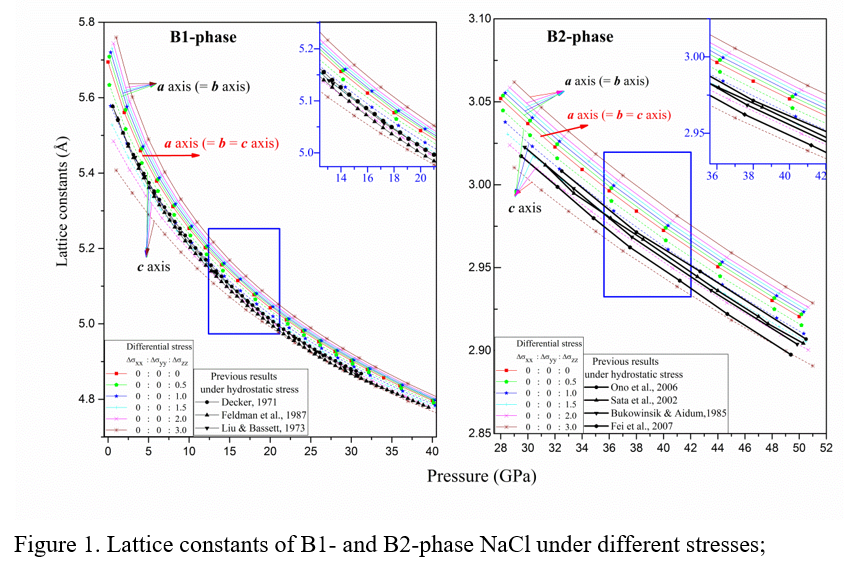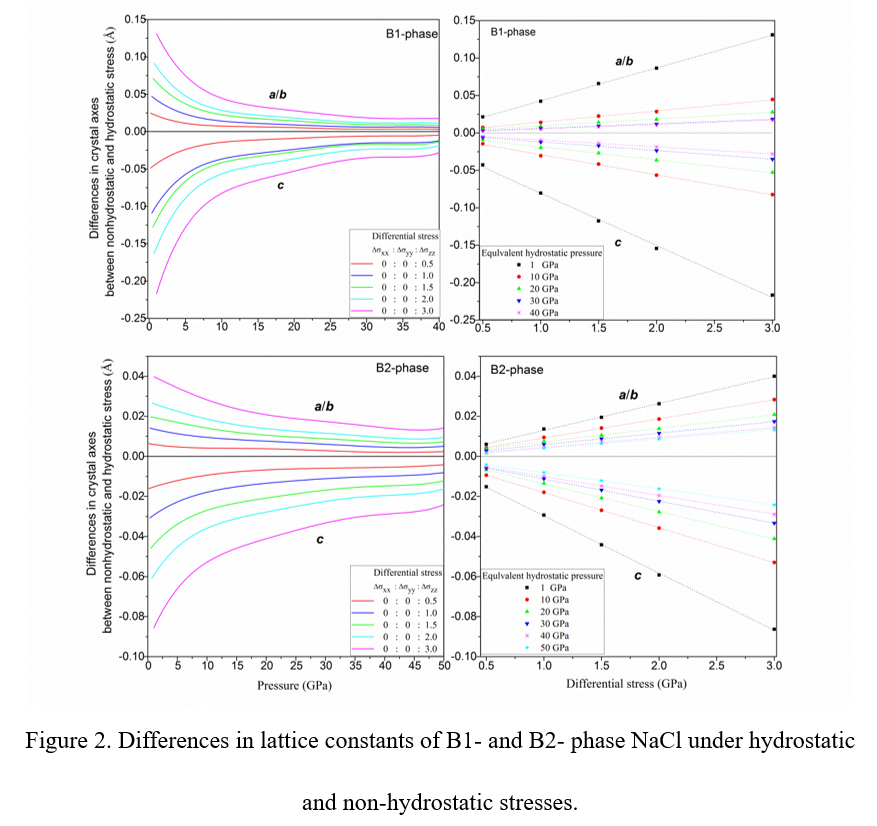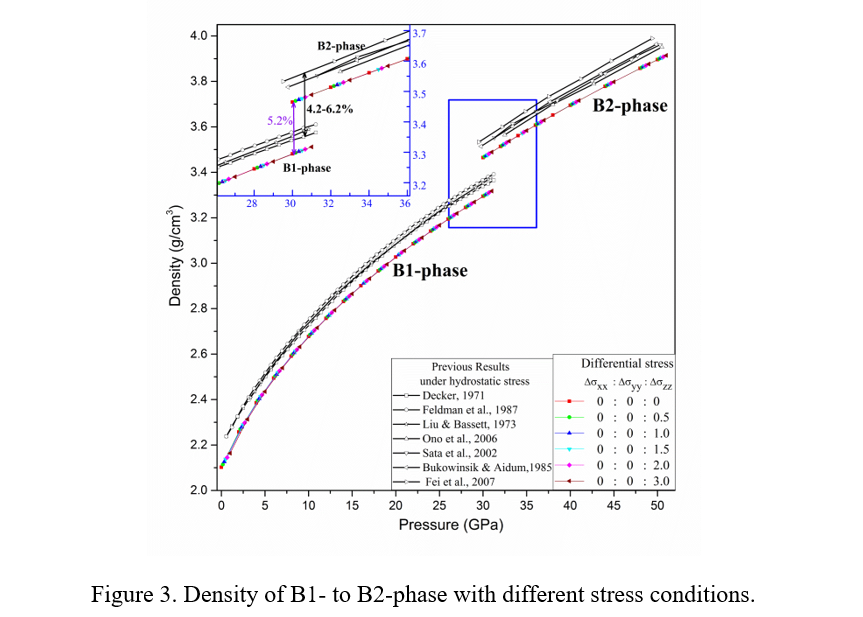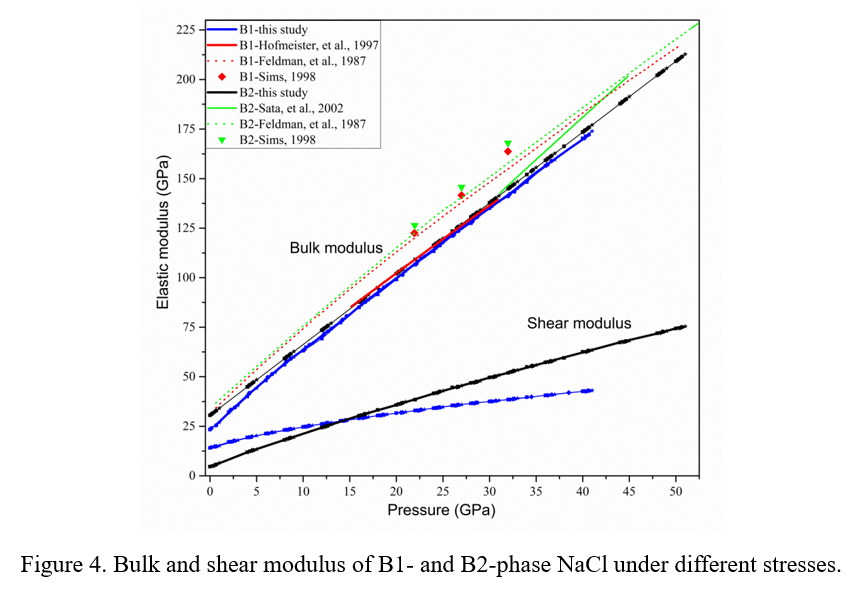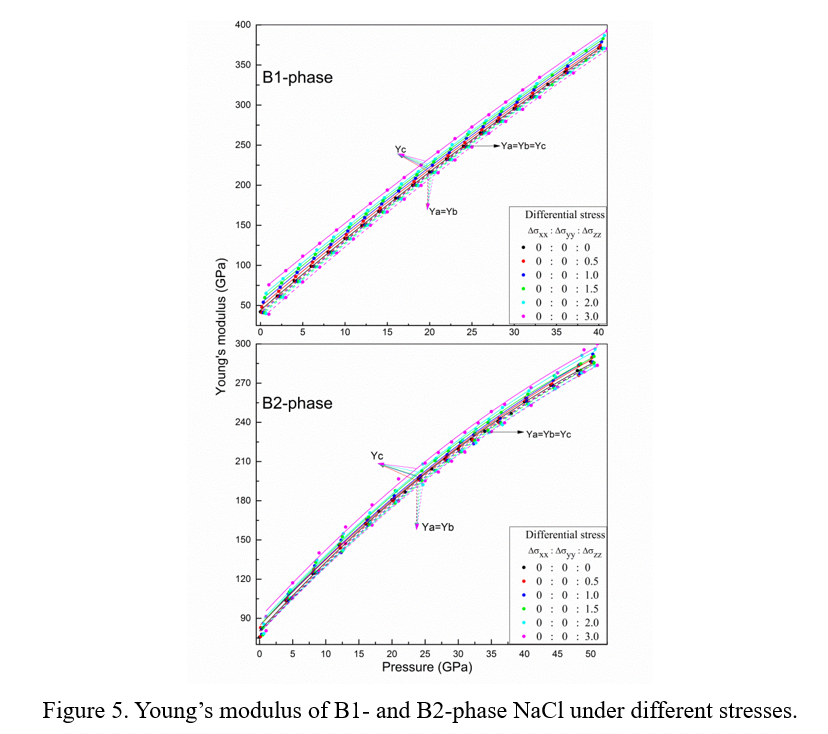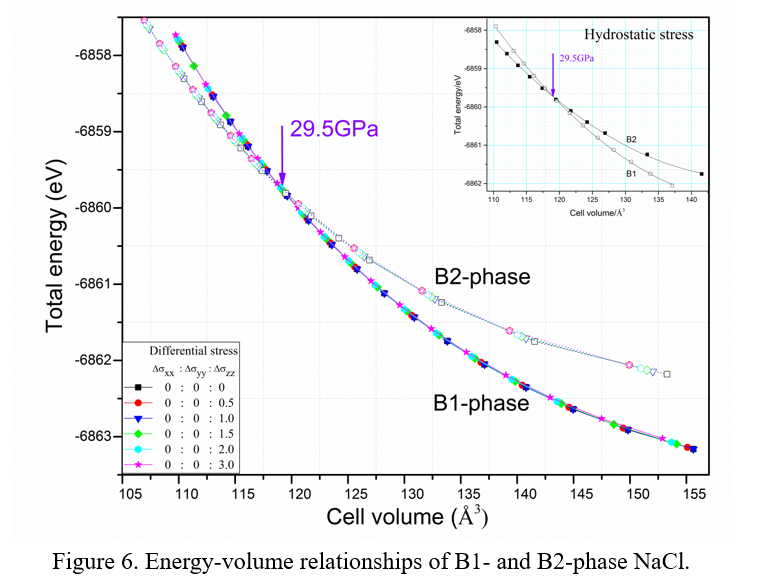Effect of non-hydrostatic stress on the structure and elasticity of NaCl by first-principles calculation

AIP Advances 9, 055014 (2019); https://doi.org/10.1063/1.5100916

liulei@ief.ac.cn (L. Liu)

Non-hydrostatic stresses have a unique influence on materials. To understand the effect of non-hydrostatic stress on NaCl, one of the most widely used pressure calibrant in high-pressure experiments, the lattice constants, density, elastic modulus, Young’s modulus, and phase transitions of the B1 and B2 phases of NaCl were calculated under hydrostatic and non-hydrostatic stresses. The effects of non-hydrostatic stresses on the lattice constants and Young’s modulus show the Poisson effect. The effect of non-hydrostatic stresses on lattice constants increases linearly with increasing differential stress, however, these effects decrease with increasing pressure. Non-hydrostatic stresses explored in this study have no effect on the density (the equation of state), elastic modulus, and phase-equilibrium pressure of the B1 and B2 phases of NaCl). Therefore, non-hydrostatic stresses do not affect the NaCl acting as a pressure scale to indicate pressure values in high-pressure experiments, but it may not accurately reflect the state of stress. The state of non-hydrostatic stress is complex in high-pressure experiments, but we only considered one non-hydrostatic state in this study; thus, more work is needed to clarify the effect of non-hydrostatic stress.

Keywords: Non-hydrostatic stress, NaCl, Crystal structure and elasticity, First-principles calculation.